﻿ Effect of Mesoscale Land Use Change on Characteristics of Convective Boundary Layer: Semi-Idealized Large Eddy Simulations over Northwest China
 J. Meteor. Res.2018, Vol. 32Issue (3): 421-432PDF
http://dx.doi.org/10.1007/s13351-018-7185-8
The Chinese Meteorological Society
0

#### Article Information

CAO, Bangjun, Shuwen ZHANG, Deqin LI, et al., 2018.
Effect of Mesoscale Land Use Change on Characteristics of Convective Boundary Layer: Semi-Idealized Large Eddy Simulations over Northwest China. 2018.
J. Meteor. Res., 32(3): 421-432
http://dx.doi.org/10.1007/s13351-018-7185-8

### Article History

Received July 1, 2017
in final form January 7, 2018
Effect of Mesoscale Land Use Change on Characteristics of Convective Boundary Layer: Semi-Idealized Large Eddy Simulations over Northwest China
Bangjun CAO1,2, Shuwen ZHANG2, Deqin LI3, Yanlin LI2, Linfan ZHOU2, Jiemin WANG4
1. School of Atmospheric Sciences, Chengdu University of Information Technology, Chengdu 610225;
2. Key Laboratory for Semi-Arid Climate Change of the Ministry of Education, College of Atmospheric Sciences, Lanzhou University, Lanzhou 730000;
3. Shenyang Central Meteorological Observatory, Shenyang 110016;
4. Cold and Arid Regions Environmental and Engineering Research Institute, Chinese Academy of Sciences, Lanzhou 730000
ABSTRACT: Although large-scale topography and land use have been properly considered in weather and climate models, the effect of mesoscale and microscale heterogeneous land use on convective boundary layer (CBL) has not been fully understood yet. In this study, the influence of semi-idealized strip-like patches of oases and deserts, which resemble irrigated land use in Northwest China, on the CBL characteristics, is investigated based on the Weather Research and Forecasting (WRF)-large eddy simulation (LES) driven by observed land surface data. The influences of soil water content in oases on aloft CBL flow structure, stability, turbulent kinetic energy (TKE), and vertical fluxes are carefully examined through a group of sensitivity experiments. The results show that secondary circulation (SC)/turbulent organized structures (TOS) is the strongest/weakest when soil water content in oases is close to saturation (e.g., when the oases are irrigated). With the decrease of soil water content in oases (i.e., after irrigation), SC (TOS) becomes weak (strong) in the lower and middle CBL, the flux induced by SC and TOS becomes small (large), which has a dramatic impact on point measurement of eddy covariance (EC) fluxes. The flux induced by SC and TOS has little influence on EC sensible heat flux, but great influence on EC latent heat flux. Under this circumstance, the area averaged heat flux cannot be represented by point measurement of flux by the EC method, especially just after irrigation in oases. Comparison of imbalance ratio (i.e., contribution of SC and TOS to the total flux) reveals that increased soil moisture in oases leads to a larger imbalance ratio as well as enhanced surface heterogeneity. Moreover, we found that the soil layer configuration at different depths has a negligible impact on the CBL flux properties.
Key words: oasis and desert     land surface heterogeneity     large eddy simulation     soil water content     secondary circulation     turbulent organized structure
1 Introduction

Land surface heterogeneities may result from topography and land use, ranging from continental scale down to mesoscale and microscale. Although large-scale topography and land use have been properly considered in weather and climate models, the effect of mesoscale and microscale heterogeneous land use on convective boundary layer (CBL) has not been fully understood yet, especially for microscale heterogeneous land use (Heinemann and Kerschgens, 2005).

Because an atmospheric general circulation model (GCM) or a mesoscale weather model is not able to correctly resolve microscale physical processes resulted from microscale topographical and land use heterogeneities, turbulence-resolving large eddy simulation (LES) has been used to investigate the interaction between heterogeneous land surface and the atmosphere aloft (Shen and Leclerc, 1995; Raasch and Harbusch, 2001; Courault et al., 2007; Huang and Margulis, 2010; Shen et al., 2016). For example, Avissar and Schmidt (1998) showed that secondary circulation (SC) was strong when the amplitude and wavelength of an ideal surface heat wave were large; Maronga and Raasch (2013) investigated the impact of real surface heterogeneity on CBL and found the developments of SC with local or roll-like patterns.

To better investigate the interaction between land surface heterogeneity and the atmosphere aloft, LES has been coupled to land surface model (LSM) (Courault et al., 2007; Huang and Margulis, 2010; Shao et al., 2013). For example, Patton et al. (2005; hereafter PSM05) reported a large contribution of SC to vertical fluxes by using an idealized one-dimensional soil moisture heterogeneity, and Shao et al. (2013) investigated the impact of real surface heterogeneities on SC and found that the heterogeneity induced fluxes were closely related to land use patterns.

In the arid region of northwestern China, a specific type of land surface exists with a mixture of Gobi, sand desert, and oasis (Liu et al., 2011; Wang et al., 2012), where irrigation has been widely adopted (Li et al., 2013). Adding soil water into oases further enlarges the difference of land surface physical characteristics between oases and surrounding deserts. Up to now, few studies have been carried out to investigate the influence of this kind of surface heterogeneity on flow structures and vertical fluxes in the CBL with the coupled LES.

In addition, LES has been adopted to investigate point flux underestimation by the eddy covariance (EC) method (Steinfeld et al., 2007; Foken, 2008; Huang et al., 2008; Wang et al., 2009; Foken et al., 2010). Kanda et al. (2004) and Inagaki et al. (2006) demonstrated that SC with the scale between 4 and 16 km and local advection by turbulent organized structures (TOS) with the scale of 1–2 km or less were both causes for the flux underestimation by EC.

In this study, the CBL structure over semi-idealized strip-like patches of deserts and oases is first investigated; the effect of soil water content in oases on CBL flow structure is analyzed; the contribution of SC as well as TOS to energy balance is quantified; and the effect of soil layer configuration on CBL characteristics is finally examined. This paper is organized as follows. Section 2 describes the LES model and associated experimental design. Section 3 describes the analysis method. The simulation results and discussion are presented in Section 4. A summary is provided in Section 5.

2 Introduction of models and experimental design 2.1 Models

The Weather Research and Forecasting (WRF) model in its large eddy simulation (WRF-LES) coupled to the Community Noah land surface model with multi parameterization options (Noah-MP) was used (Moeng et al., 2007; Niu et al., 2011). The WRF-LES has state-of-the-art numerical schemes (e.g., higher-order time and advection schemes), and has been evaluated and validated over different heterogeneous lands (Solomon et al., 2011; Talbot et al., 2012; Yamaguchi and Feingold, 2012). Talbot et al. (2012) showed that simulation with the fine resolution WRF-LES produced better surface fluxes and near-surface meteorological parameters.

In our simulations, subgrid scale (SGS) turbulence parameterization is 1.5-order TKE closure scheme (Klemp and Wilhelmson, 1978; Deardorff, 1980). Lateral boundaries are periodic condition. Surface layer scheme is based on Monin–Obukhov (MO) similarity theory. A 2-m depth of soil is divided into 4 layers with thicknesses of 0.1, 0.3, 0.6, and 1.0 m, respectively. The chosen parameterization schemes are ball-berry canopy stomatal resistance scheme, MO surface layer drag coefficient scheme, modified two-stream radiation transfer scheme, and CLASS (Canadian Land Surface Scheme) ground snow surface albedo scheme.

2.2 Experimental design

The horizontal domain in this study covers an area of 20 km × 5 km with Δx = Δy = 50 m, and vertical height is 2.6 km. The variable Δz changes from 10 m near the surface to 40 m for z ≥ 100 m. Time step is 0.4 s. A Rayleigh damping layer is set to damp the reflection of gravity waves (Klemp et al., 2008), and the thickness of the damping layer is 500 m. Initial potential temperature and moisture profiles for LES are taken from radiosonde measurements at 1200 local time (LT) 1 August 2012 at Zhangye (39°5′15″N, 100°16′39″E, 1556.06 m a.s.l.) (Li et al., 2013). Initial potential temperature is 310 K below 925 m, increases by 8 K over a strong inversion depth of 150 m; initial CBL height zi is 1000 m, and above 1000 m, stratification is 3 K km–1; initial water vapor mixing ratio is constant, with a value of 7 g kg–1 below 900 m, dropping sharply to 2 g kg–1 across initial inversion layer between 925 and 1075 m; initial horizontal mean wind is zero for all cases.

Incoming solar radiation is 800 W m–2 and longwave solar radiation is 350 W m–2 by averaging measurements at Daman (38°51′19″N, 100°22′20″E, 1556.06 m a.s.l.) in the oasis patch between 1000 and 1600 LT during the study period, respectively. Atmospheric radiation scheme is not included so any longwave radiation leaving the surface and scattered shortwave radiation are assumed to radiate into space. Albedo is diagnosed from the surface solar radiation budget.

The LES is run with land use conditions supplied from the LSM outputs. In the LSM simulations, the strip-like land types of oasis and desert are specified as cropland/woodland mosaic and barren as in the United States Geological Survey, respectively, with no variation in the y-direction (Fig. 1). Roughness length is obtained from a lookup table; the minimum and maximum background roughness lengths are 5 and 14 cm for oasis, respectively, and 1 and 1 cm for desert. The coupled LES–LSM simulations are carried out on 4 representative days of 1, 8, 15, and 21 August, representing the 3rd, 10th, 17th, and 23rd days (hereafter referred as D03, D10, D17, and D23) after irrigation, and each simulation was integrated for 5 h. Initial soil water contents in oasis and desert on the above 4 days are taken from measurements at 1200 LT at Daman station and Shenshawo desert site (38°47′21″N, 100°29′35″E, 1594.00 m a.s.l.), respectively (Table 1 and Fig. 2). Initial soil temperatures are based on ground-based measurements at Daman and Shenshawo at 1200 LT 1 August in all cases (Table 2).

Eleven coupled simulation experiments are conducted (see Table 3). They are named as follows. D03-10S, for example, refers to a simulation on the 3rd day after irrigation with the width (i.e., λ) of one patch of oasis and desert equal to 10 km. In the first two cases (D03-OS and D03-DS, Table 3), only one type of land surface, i.e., either oasis (OS) or desert (DS), is used with no land heterogeneity. D03-10SA is the same as D03-10S except for using a different soil layer configuration.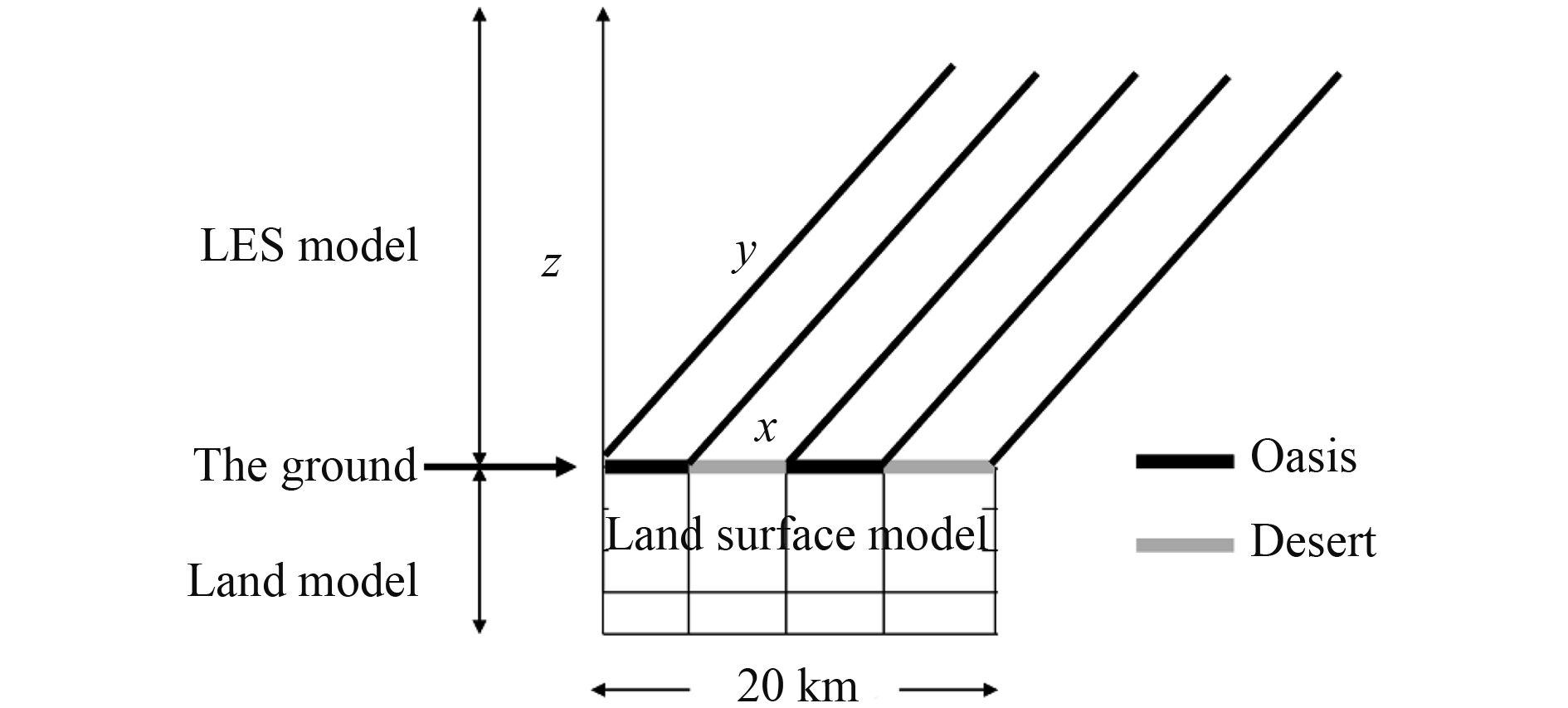Figure 1 Schematic diagram showing the coupling of the LES model to the LSM.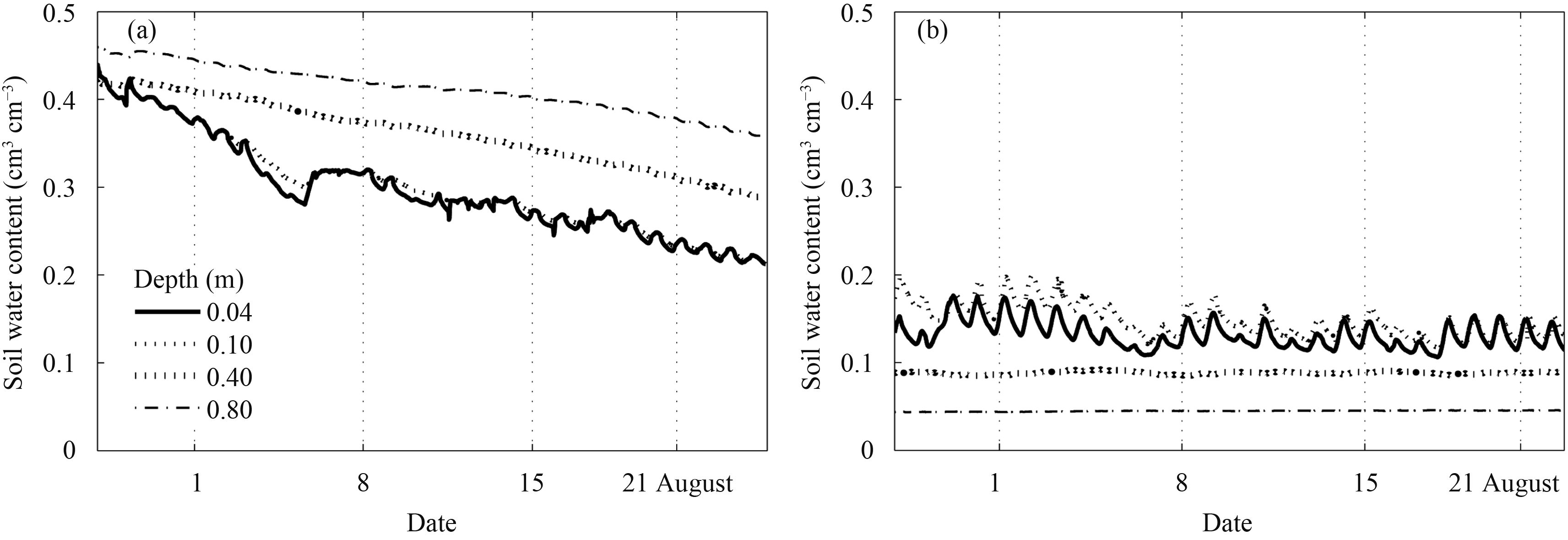Figure 2 Temporal evolutions of soil water content at four different depths (0.04, 0.10, 0.40, and 0.80 m) from 1 to 25 August (irrigation was carried out on 29 July) at observation sites of (a) Daman and (b) Shenshawo.
Table 1 Initial soil water content (cm3 cm–3) in the four soil layers of the LSM, prescribed as the observed values at Daman (representing oasis) and Shenshawo (representing desert)
 Oasis Desert Layer 1 Layer 2 Layer 3 Layer 4 Layer 1 Layer 2 Layer 3 Layer 4 D03 0.38 0.39 0.41 0.42 0.15 0.15 0.092 0.05 D10 0.32 0.32 0.39 0.41 0.15 0.15 0.092 0.05 D17 0.28 0.28 0.35 0.41 0.15 0.15 0.092 0.05 D23 0.27 0.27 0.35 0.39 0.15 0.15 0.092 0.05
Table 2 Initial soil temperature (K) in the four layers of the LSM, prescribed as the observed values at Daman (representing oasis) and Shenshawo (representing desert)
 Oasis Desert Layer 1 Layer 2 Layer 3 Layer 4 Layer 1 Layer 2 Layer 3 Layer 4 D03 292.1 292.1 291.9 291.6 296.8 297.2 296.4 295.5 D10 292.1 292.1 291.9 291.6 296.8 297.2 296.4 295.5 D17 292.1 292.1 291.9 291.6 296.8 297.2 296.4 295.5 D23 292.1 292.1 291.9 291.6 296.8 297.2 296.4 295.5
Table 3 Basic properties for various cases
 Case λ (km) zi (km) $[\overline H ]$ (W m–2) $[\overline {{\rm{LE}}} ]$ (W m–2) w* (m s–1) Oasis Desert $[\overline H ]$ $[\overline {{\rm{LE}}} ]$ $[\overline H ]$ $[\overline {{\rm{LE}}} ]$ D03-OS – 1.10 50.99 331.56 0.82 – – – – D03-DS – 1.25 300.72 80.23 2.51 – – – – D03-10S 10 1.16 180.67 202.34 2.01 50.14 352.28 310.22 51.42 D03-10SA 10 1.32 198.67 184.56 2.26 85.83 315.62 311.34 53.56 D03-20S 20 1.15 179.08 200.68 2.02 45.56 350.76 312.13 51.68 D10-10S 10 1.17 201.23 172.24 2.26 91.26 292.08 313.24 52.41 D10-20S 20 1.16 202.94 171.51 2.23 93.28 292.42 311.63 51.16 D17-10S 10 1.18 224.39 141.34 2.37 137.58 230.28 313.27 52.82 D17-20S 20 1.17 226.43 139.38 2.33 140.26 228.16 312.96 50.64 D23-10S 10 1.21 249.14 112.67 2.43 187.08 172.94 311.86 52.43 D23-20S 20 1.20 250.68 111.45 2.41 188.76 172.31 312.72 51.67 Note: λ: width of one patch of oasis and desert; zi: CBL height; $[\overline H ]$ : sensible flux; $[\overline {{\rm{LE}}} ]$ : latent heat flux; w*: convective velocity scale. D03-10SA is the same as D03-10S except for using a different soil layer configuration.
3 Description of methods

Under a few assumptions such as no synoptic ascending/descending motion, flat ground surface, no background horizontal wind, and periodic boundary condition, (total) vertical flux can be decomposed into the EC flux, SC flux, and TOS flux (Inagaki et al., 2006). Furthermore, to quantify the contribution of SC and TOS to the total flux, horizontal domain average of the imbalance ratio [I] (which can be decomposed into [Ih] for sensible heat flux and [Iq] for latent heat flux) and phase average of the imbalance ratio [I]p are defined as

 $[I] = ([{[\overline w ]_{\rm p}}{[\overline \varphi ]_{\rm p}}] + [\overline w _{\rm p}'\overline \varphi _{\rm p}'])/[\overline {w\varphi } ],$ (1)
 ${[I]_{\rm p}} = {[([\overline {w\varphi } ] - \overline {w_{\rm t}'\varphi _{\rm t}'})/[\overline {w\varphi } ]]_{\rm p}},\quad\,\,\,$ (2)

where w is vertical velocity (positive upward) and φ is a scalar variable like potential temperature θ and specific humidity q; prime with subscript t or p means temporal fluctuation or temporal-plus-phase fluctuation; overbar indicates temporal averaging; [ ] and [ ]p are horizontal domain average and phase average, respectively. The variable $[\overline {w\varphi } ]$ is total flux, $\overline {w_{\rm t}'\varphi _{\rm t}'}$ is EC flux, ${[\overline w ]_{\rm p}}{[\overline \varphi ]_{\rm p}}$ is resolved SC flux (hereafter referred to as SC), and $[\overline w _{\rm p}'\overline \varphi _{\rm p}']$ is the resolved TOS flux (hereafter referred to as TOS). In the analysis, the EC is evaluated as the sum of computed grid-scale (GS) and subgrid-scale (SGS) components. See Appendix for details.

To acquire the quasi-steady state of the developing CBL, data analysis is carried out after 3.5-h integration. For all statistics, the averaging time is 1 h, which is a typical value used in field observations.

For normalization and comparison purposes, Table 3 lists basic properties of different cases, such as the width of one patch of oasis and desert (λ), CBL height (zi), total horizontal averaged surface sensible flux ( $[\overline H ]$ ), and latent heat flux ( $[\overline {{\rm{LE}}} ]$ ), and convective velocity scale ${w_*}{\rm{ = [(}}g/\rho){z_i}{\rm{([}}\overline H ]/({\theta _0}{c_p}){\rm{ + 0}}{\rm{.61[}}\overline E {\rm{])}}{{\rm{]}}^{1/3}}$ , where g is the gravitational acceleration, θ0 is reference temperature, $[\overline E ]$ is total surface latent heat flux, θv* is virtual potential temperature scale, and q* is humidity scale.

4 Results 4.1 CBL flow structure and atmosphere stability

To illustrate the influence of strip-like oasis and desert patches on CBL flow structure, Fig. 3 shows the distributions of vertical velocity and water vapor mixing ratio averaged over 3.5–4.5-h integration for D03-10S. The ascending motion centers are near the middle of deserts at x = 7.5 and 17.5 km, confined to 30% of the wavelength, with a weak descending motion elsewhere (Fig. 3a). Over oases, low water vapor zone in the upper CBL extends to the middle CBL due to the entrained dry air. In the lower CBL, water vapor mixing ratio over the oasis side is much larger than that over the desert side (Fig. 3b).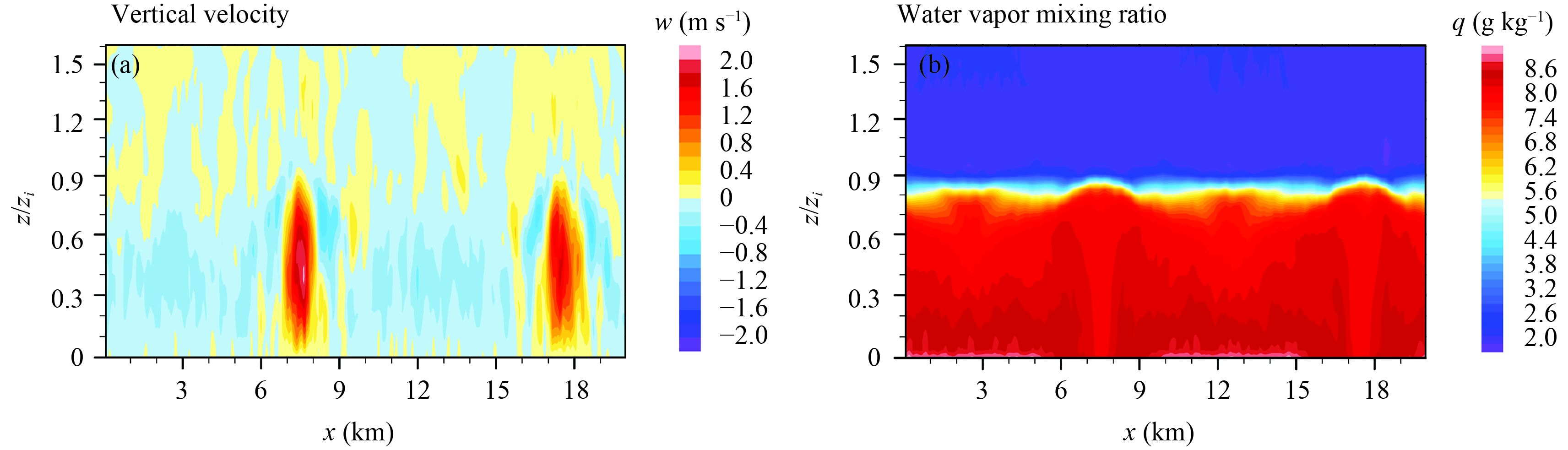Figure 3 Snap shots of (a) vertical velocity and (b) water vapor mixing ratio, averaged along the y-axis of the study domain, after 3.5-h integration for D03-10S.

To have a clear picture of vertical flow at different scales, temporal mean vertical velocity $\overline w$ at the height of 100 m (Figs. 4a, b) is decomposed into SC part ( ${[\overline w ]_{\rm p}}$ ) (Figs. 4c, d) and TOS part ( $[\overline w ] - {[\overline w ]_{\rm p}}$ ) (Fig. 4e, f). For a better resolution, only one oasis–desert heterogeneity cycle is depicted. Figures 4a and 4b show that ascending motion is centered over the middle of desert for D03-10S, but is not clear for D23-10S; on the contrast, the “rib-like” pattern of $\overline w$ for D23-10S is more obvious than that for D03-10S. Spatial structure of ${[\overline w ]_{\rm p}}$ (Figs. 4c, d) has the same one-dimensional variation as surface heterogeneity and $\overline w$ (Figs. 4a, b). The maximum of ${[\overline w ]_{\rm p}}$ occurs at 7.5 km, the minimum is at 2.5 km, and their difference is more than 0.6 m s–1 for D03-10S with an obvious SC (Figs. 4c), but less than 0.3 m s–1 for D23-10S with no clear SC (Figs. 4d). As for TOS ( $[\overline w ] - {[\overline w ]_{\rm p}}$ ), a very complicated “rib-like” pattern appears for both D03-10S and D23-10S (Figs. 4e, f), especially for D23-10S (Figs. 4f).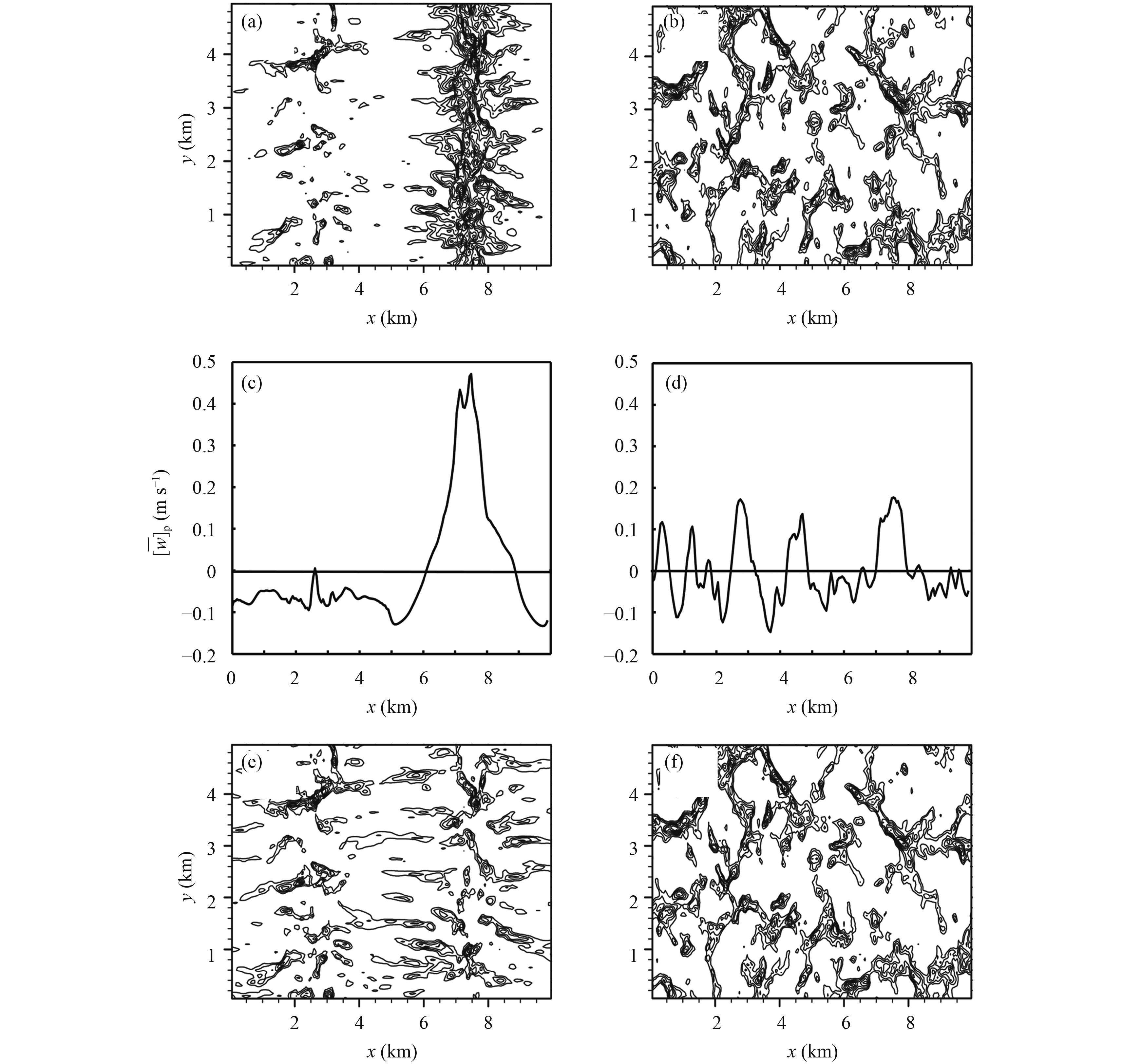Figure 4 Temporal averages of vertical velocity ( $\overline w$ ) for (a) D03-10S and (b) D23-10S, and TOS ( $[\overline w ] - {[\overline w ]_{\rm p}}$ ) for (e) D03-10S and (f) D23-10S. The SC ( ${[\overline w ]_{\rm p}}$ ) averaged along the y-axis of the domain for (c) D03-10S and (d) D23-10S. Values in all panels are at the height of 100 m, and the contours range from 0.1 to 0.6 m s–1 at an interval of 0.1 m s–1 in (a, b, e, f).

To quantitatively compare the variation of SC and TOS with the decrease of soil water content over oases from the 3rd to 23rd days after irrigation, the phase averaging standard deviation of $\overline w$ is calculated as

 ${\sigma _w}(\overline x) = \sqrt {{{({N_{\rm p}}{N_y})}^{ - 1}}\sum\limits_{n = 1}^{{N_{\rm p}}} {\sum\limits_{y = 1}^{{N_y}} {{{[\overline w (\overline x + n\lambda, y) - {{[\overline w ]}_{\rm p}}]}^2}} } } ,$ (3)

where ${\sigma _w}(\overline x)$ is the strength of temporally averaging TOS (Inagaki et al., 2006). At the height of 100 m, ${\sigma _w}(\overline x)$ over the center of the oasis or desert is larger than that at the edge of the oasis or desert for D03-10S (Fig. 5a). Although no large-scale background horizontal wind exists, the heterogeneous surface induces horizontal wind across the inner domain of oasis and desert for D03-10S (Fig. 5b). With the decrease of soil water content in the oases, TOS increases, and thus, ${\sigma _w}(\overline x)$ becomes larger at all locations and heights within the CBL (figure omitted).

To illustrate the impact of soil water content in oases on the aloft atmosphere stability, the x-directional distribution of gradient Richardson number (Ri) at 100-m height, defined by

 ${\rm{Ri}} = (g/\theta)(\partial \theta /\partial z)/{(\partial u/\partial z)^2},$ (4)

is shown in Fig. 5c. In all cases, background Ri is equal to 0. But flow becomes dynamically stable with Ri > 0.25 over oases and very unstable with Ri < 0 over deserts as in the case of D03-10S. When oases continue to become dry as in the case of D23-10S, flow becomes very dynamically unstable with Ri < 0 over both oases and deserts. In a word, the atmosphere stability over oases and deserts is different just after irrigation, but with the decrease of soil water content in oases, the stability over oases and deserts becomes similar.

Total turbulent kinetic energy (TKE) (both domain and time averaged over the CBL normalized by w*2) responds sensitively to land surface heterogeneity; for example, TKE is 0.51 just after irrigation in oases, but it rises to 0.68 on the 23rd day, due to the increased sensible heat flux.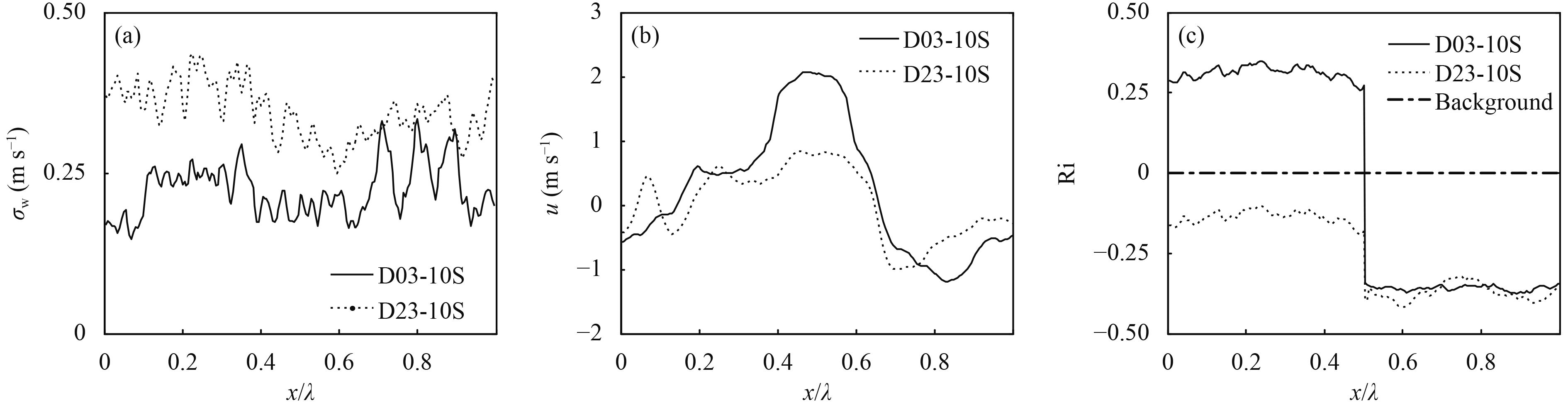Figure 5 (a) Standard deviation of temporal average of vertical velocity, (b) temporal average of velocity in the x-direction, and (c) Ri at the height of 100 m (dot-dashed line is background atmosphere Ri).

To investigate dependence of the intensity of SC on soil water content in oases, correlation analysis between surface heat flux and vertical velocity is applied (Maronga and Raasch, 2013). A high correlation between ${[\overline H ]_{\rm p}}$ and ${[\overline w ]_{\rm p}}$ exists, especially at low levels, and becomes smaller with the decrease of soil water content in oases (Table 4). Besides, correlation coefficient decreases with the increase of height, indicating that the influence of oasis–desert patterns decreases with height.

Table 4 Correlation coefficient between ${[\overline H ]_{\rm p}}$ and ${[\overline w ]_{\rm p}}$ at different heights
 z/zi D03-10S D23-10S Oasis Desert Oasis Desert 0.1 0.73 0.81 0.63 0.73 0.2 0.56 0.65 0.55 0.62 0.3 0.44 0.51 0.42 0.46 0.4 0.32 0.39 0.25 0.31
4.2 Vertical fluxes in the CBL 4.2.1 Vertical fluxes

Figure 6a shows the normalized profiles of total sensible heat flux ( $[\overline {w\theta } ]$ ), EC sensible heat flux ( $[\overline {w_{\rm t}'\theta _{\rm t}'} ]$ ), SC sensible heat flux ( $[{[\overline w ]_{\rm p}}{[\overline \theta ]_{\rm p}}]$ ), and TOS sensible heat flux ( $[\overline w _{\rm p}'\overline \theta _{\rm p}']$ ) for D03-10S, and the corresponding latent heat fluxes are shown in Fig. 6b. Both total sensible heat flux ( $[\overline {w\theta } ]$ ) and total latent heat flux ( $[\overline {wq} ]$ ) display classical profiles as shown by Deardorff (1972); total sensible heat flux decreases linearly with height and even becomes negative in entrainment zone (Fig. 6a), and total latent heat flux increases with height up to the entrainment level and then quickly reduces to zero (Fig. 6b).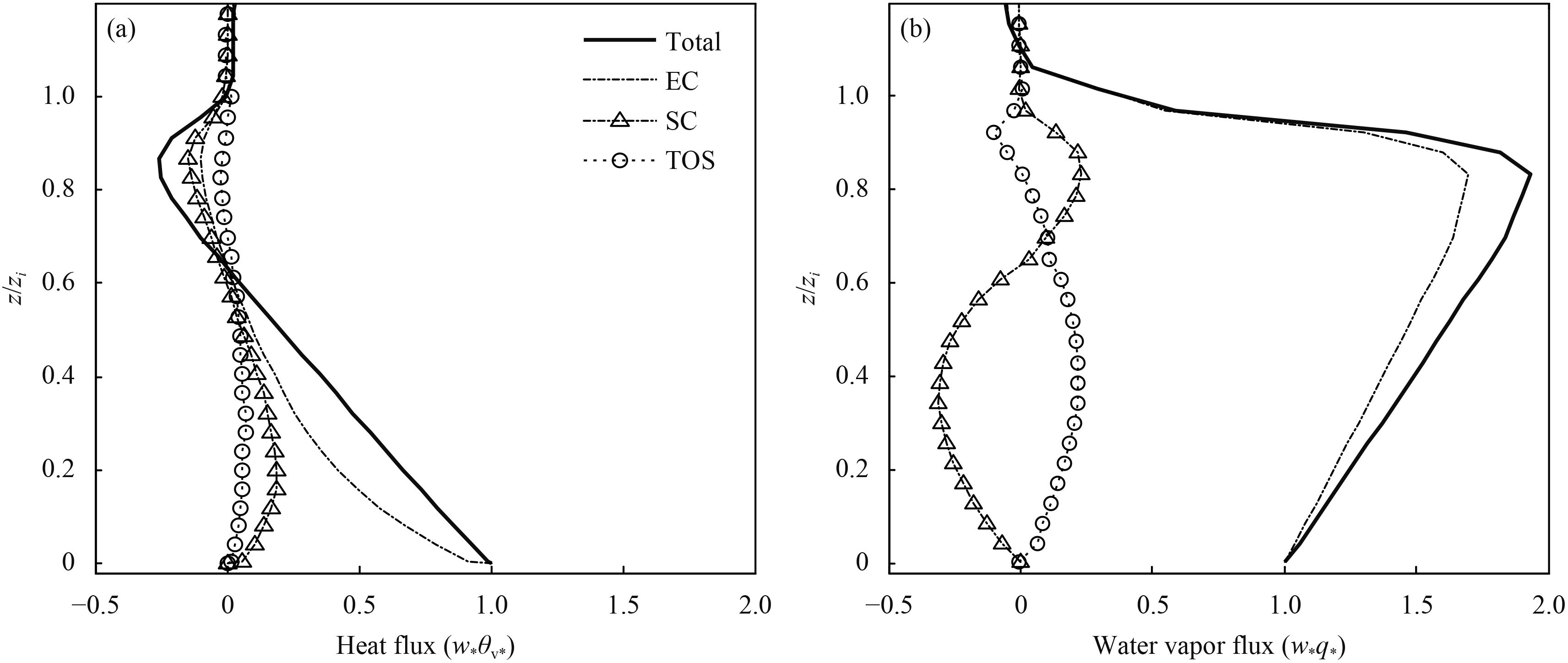Figure 6 Comparison of normalized total flux, EC, SC, and TOS for (a) sensible heat and (b) latent heat fluxes for case D03-10S.

In the CBL, EC sensible heat flux ( $[\overline {w_{\rm t'}\theta _{\rm t}'} ]$ ) dominates total sensible heat flux but decreases with height (Fig. 6a). SC sensible heat flux ( $[{[\overline w ]_{\rm p}}{[\overline \theta ]_{\rm p}}]$ ) first increases with height, reaches the maximum of 0.22 at z/zi = 0.25, and then decreases. TOS sensible heat flux ( $[\overline w _{\rm p}'\overline \theta _{\rm p}']$ ) has a very slight variation with height. Relatively speaking, SC sensible heat flux is larger than TOS flux at the lower CBL.

Normalized EC latent heat flux ( $[\overline {w_{\rm t}'q_{\rm t}'} ]$ ) reaches the maximum of 1.72 at z/zi = 0.85 (Fig. 6b). SC latent heat flux ( $[{[\overline w ]_{\rm p}}{[\overline q ]_{\rm p}}]$ ) is negative with the minimum of –0.19 at z/zi = 0.30, and becomes positive in the upper CBL above z/zi > 0.60. The contribution of TOS latent heat flux ( $[\overline w _{\rm p}'\overline q _{\rm p}']$ ) to the total flux is positive, with the maximum of 0.34 at z/zi = 0.30. Because of the strong descending motion of SC and relative larger water vapor mixing ratio over oases than over deserts, SC latent heat flux is downward below z/zi = 0.60 and thus water vapor at low levels is prevented from transporting into the atmosphere aloft over the oasis (Fig. 3b) (Chu et al., 2005; Meng et al., 2012, 2015). Because of different experimental settings from our study, previous studies have not reported negative SC latent heat flux (e.g., Inagaki et al., 2006).

4.2.2 Effect of soil water content on vertical fluxes

To show the effect of different soil water contents on vertical fluxes, the normalized profiles of the total vertical advection flux (i.e., the sum of SC and TOS), and the SC and TOS for D03-10S and D23-10S are plotted in Fig. 7 for sensible heat and in Fig. 8 for latent heat (water vapor). With the decrease of soil water content in oases, total vertical advection sensible heat flux decreases from the maximum of 0.26 in D03-10S to 0.20 in D23-10S at z/zi = 0.25 (Fig. 7a), SC sensible heat flux at z/zi = 0.25 decreases significantly from the maximum of 0.22 in D03-10S to 0.06 in D23-10S (Fig. 7b), and TOS sensible heat flux increases from the maximum of only 0.04 at z/zi = 0.20 in D03-10S to 0.14 in D23-10S (Fig. 7c). In a word, SC becomes the strongest and TOS becomes the weakest when soil water content in oases is near saturation. Under this circumstance, SC is assumed to penetrate the entire CBL and exert strong influences on the CBL, and the flux induced by SC must not be ignored.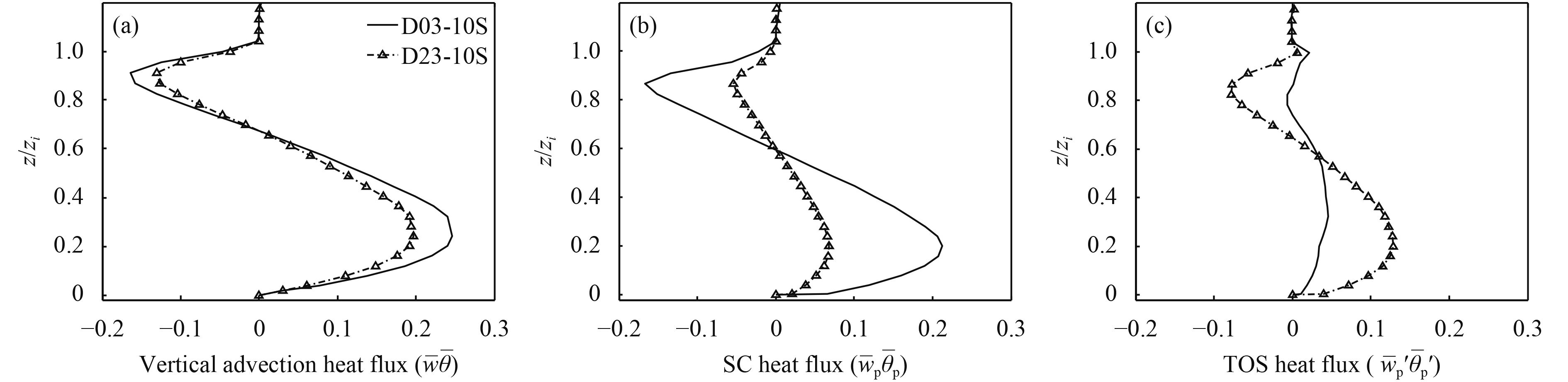Figure 7 Comparison of vertical profiles of (a) normalized vertical advection sensible heat flux ( $[\overline w \overline \theta ]$ ), (b) SC sensible heat flux ( $[{[\overline w ]_{\rm p}}{[\overline \theta ]_{\rm p}}]$ ), and (c) TOS sensible heat flux ( $[\overline w _{\rm p}'\overline \theta _{\rm p}']$ ), for cases D03-10S and D23-10S.

Different from sensible heat flux, total vertical advection flux for water vapor increases from 0.28 at z/zi = 0.85 in D03-10S to the maximum of 0.54 in D23-10S (Fig. 8a). SC latent heat flux in all cases is downward and the absolute value of SC latent heat flux at z/zi = 0.35 decreases from 0.19 in D03-10S to 0.12 in D23-10S (Fig. 8b). TOS latent heat flux in all cases is upward and increases slightly from the maximum of 0.34 in D03-10S to 0.48 in D23-10S at z/zi = 0.43 (Fig. 8c). In a word, the decrease of soil water content in oases has a profound influence on SC and TOS with the magnitude of reduced SC flux relatively larger than that of increased TOS flux.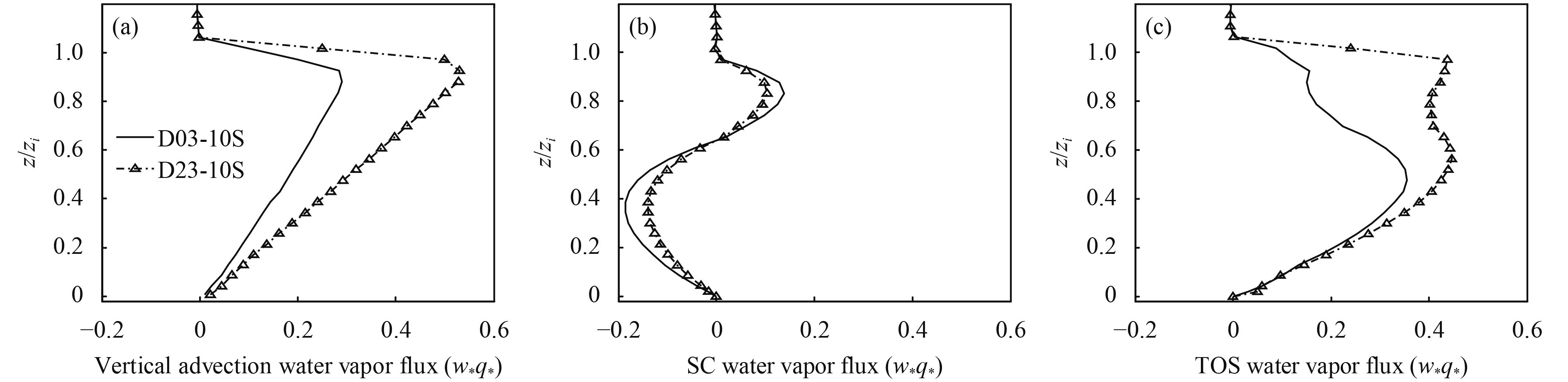Figure 8 As in Fig. 7, but for latent heat flux.

Flux patterns with λ = 20 km are similar to those with λ = 10 km, although the former flux has a slightly less magnitude (figure omitted), showing that the influence of different wave lengths on these fluxes is not significant; the reason may be related to the same atmosphere forcing used under different heterogeneity scales.

4.3 Imbalance ratio

First, we investigate the vertical variation of horizontal domain average of the imbalance ratios. The imbalance ratios for sensible heat flux [Ih] (Fig. 9a) and latent heat flux [Iq] (Fig. 10a) are about 6.1% and 1.2% at 10-m height, but rise to 24.1% and 3.2% at 100-m height, in D03-10S. Furthermore, the imbalance ratios for SC and TOS also increase with height, with the increase of magnitude for SC larger than that for TOS. For example, SC and TOS fractions of [Ih] are 3.4% and 2.7% at 10-m height, and increase to 18.3% (Fig. 9b) and 5.2% (Fig. 9c) at 100-m height, respectively.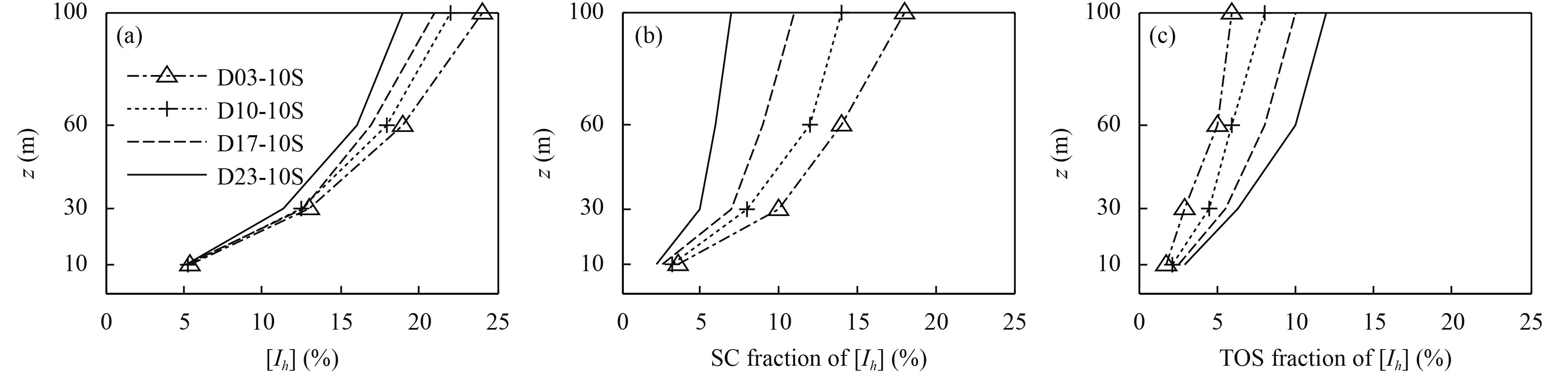Figure 9 Vertical variations of horizontal domain average of the imbalance ratios for (a) sensible heat flux, (b) SC sensible heat flux, and (c) TOS sensible heat flux.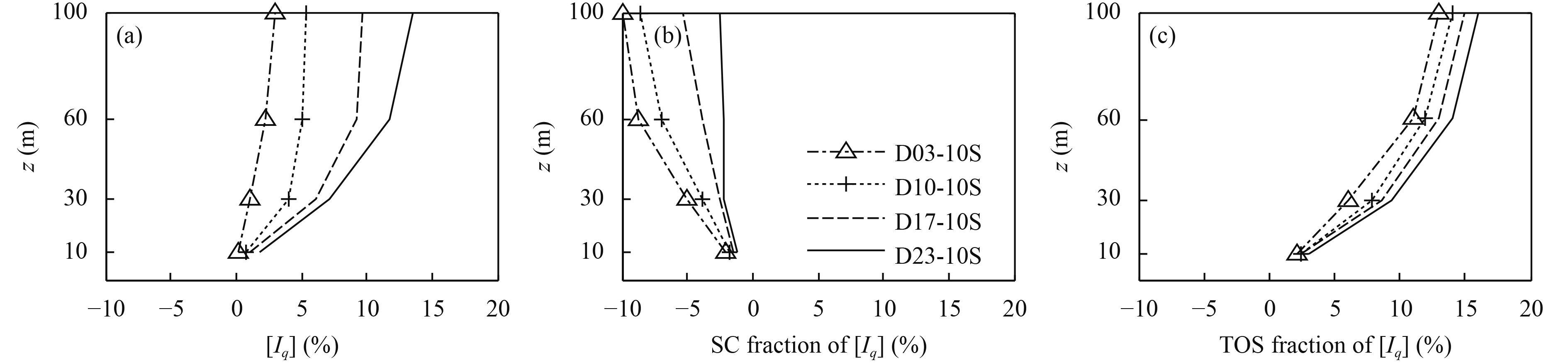Figure 10 As in Fig. 9, but for latent heat flux.

As for the impact of soil water content in oases on horizontal domain average of the imbalance ratios, the impact at low levels is very small for sensible and latent heat fluxes (Figs. 9a, 10a), SC flux (Figs. 9b, 10b), and TOS flux (Figs. 9c, 10c). At high levels (60–100 m), horizontal domain average of the imbalance ratios for sensible heat flux [Ih] and the magnitude of SC sensible heat and latent heat flux all decrease with the decrease of soil water content in oases. On the contrary, the imbalance ratios for latent heat flux [Iq], TOS sensible heat flux, and TOS latent heat flux increase. In a word, the decrease of soil water content has a significant influence on the imbalance ratio at high levels, but the influence becomes weak with the decreased height.

Second, to investigate the variation of phase average of the imbalance ratios ([Ih]p and [Iq]p), Table 5 lists their values at three x/λ locations and four levels under two soil water conditions (i.e., wet soil on the 3rd day and dry soil on the 23rd day after irrigation), respectively. The magnitude of phase average of the imbalance ratios increases with height and becomes small with oasis drying. The phase average of the imbalance ratios has a very different performance at three x/λ locations: over the center of oasis (x/λ = 0.25), the ratios always have relatively large absolute values with [Ih]p negative and [Iq]p positive, indicating that EC flux near the center of oasis has a large deviation from horizontal averaged total flux; over the joint region of oasis and desert (x/λ = 0.5), the ratio is always positive but its magnitude is relatively small; over the center of desert, [Ih]p is always positive no matter whether the oasis is wet or dry, and [Iq]p is negative but becomes relatively small with oasis drying.

Table 5 Phase average of the imbalance ratios
 z (m) Case [Ih]p (%) [Iq]p (%) x/λ= 0.25 x/λ= 0.5 x/λ = 0.75 x/λ= 0.25 x/λ= 0.5 x/λ= 0.75 10 D03-10S –45.3 20.3 45.6 72.7 25.2 –72.3 D23-10S –24.3 11.2 24.8 38.2 16.3 –38.4 30 D03-10S –48.1 24.3 47.2 74.5 30.2 –74.1 D23-10S –29.6 13.6 28.7 41.5 18.6 –41.9 60 D03-10S –50.3 27.4 48.4 75.4 32.2 –76.4 D23-10S –30.4 16.5 29.3 42.3 19.5 –42.6 100 D03-10S –53.4 30.3 50.3 76.3 33.5 –77.4 D23-10S –32.2 18.9 30.4 43.4 22.4 –45.1

Based on the above results, the mechanism for the increase of soil water content in oases on the CBL characteristics is summarized as follows. With increase of soil water content in oases, both updraft over deserts and downdraft over oases become stronger, shear (buoyant) forcing becomes weaker (stronger), and TOS (SC) becomes weaker (stronger). Meanwhile, the imbalance ratio for sensible (latent) heat flux becomes larger (smaller), and vice versa. When soil water content in oases decreases, surface heterogeneity becomes weaker and thus the difference of turbulent structure parameters between oasis and desert becomes small.

4.4 Sensitivity experiment

In the above analysis, the 2-m depth soil with fixed 4-layer thicknesses of 0.1, 0.3, 0.6, and 1.0 m was used. However, previous studies have shown that soil layer configuration had an influence on the simulation of surface heat flux and soil moisture (Zhang et al., 2009, 2010; Liu and Shao, 2013). Therefore, further experiment by using different soil layer configurations was conducted (hereafter D03-10SA), in which the default 4-layer thicknesses were respectively reduced to 0.01, 0.03, 0.06, and 0.10 m (Liu and Shao, 2013), with the rest configuration the same as D03-10S.

Compared to D03-10S, the near-surface $[\overline H ]$ is 35 W m–2 (approximately 20%) smaller and $[\overline {{\rm{LE}}} ]$ is larger by the same magnitude over oases in D03-10SA, while both $[\overline H ]$ and $[\overline {{\rm{LE}}} ]$ over deserts are almost similar (Table 3). Compared with measurements at Daman and Shenshawo observation sites, the fluxes from D03-10S are closer to the measurements than those from D03-10SA. In addition, with the decreased thickness of soil layers, soil temperature at 0.1-m depth in D03-10S is larger than that in D03-10SA (Fig. 11a), and both of them are larger than the measurements; soil water content at 0.1-m depth in D03-10S is larger than that in D03-10SA (Fig. 11b), and both of them are smaller than the measurements. Furthermore, total soil water in soil column in D03-10SA is smaller than that in D03-10S. Therefore, the increased sensible heat and decreased latent heat for D03-10SA are due to the following two factors: first, increase in soil temperature for D03-10SA causes sensible heat increase and latent heat decrease, and second, the decreased soil water in D03-10SA further makes latent heat decrease.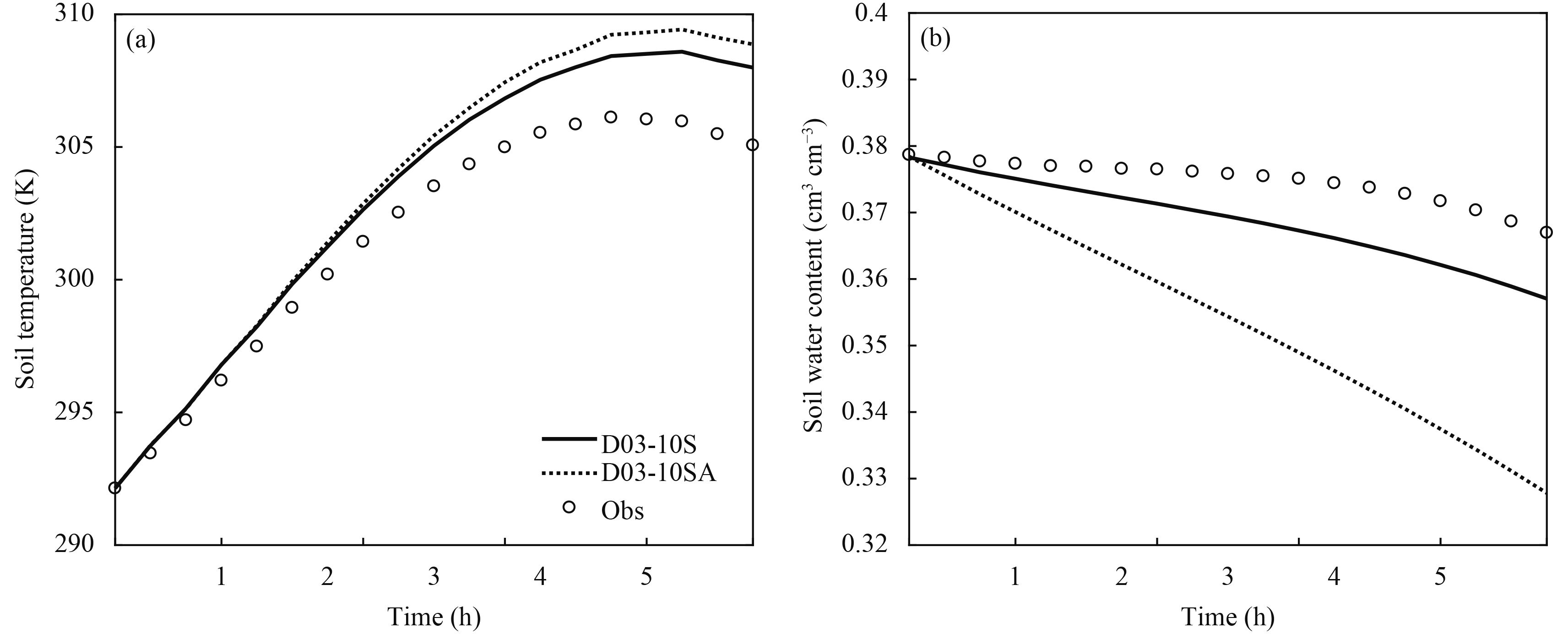Figure 11 Comparison of the simulated (a) soil temperature and (b) soil water content over oases for D03-10S (dashed line) and D03-10SA (solid line) with the observations (dots) at depth of 0.1 m.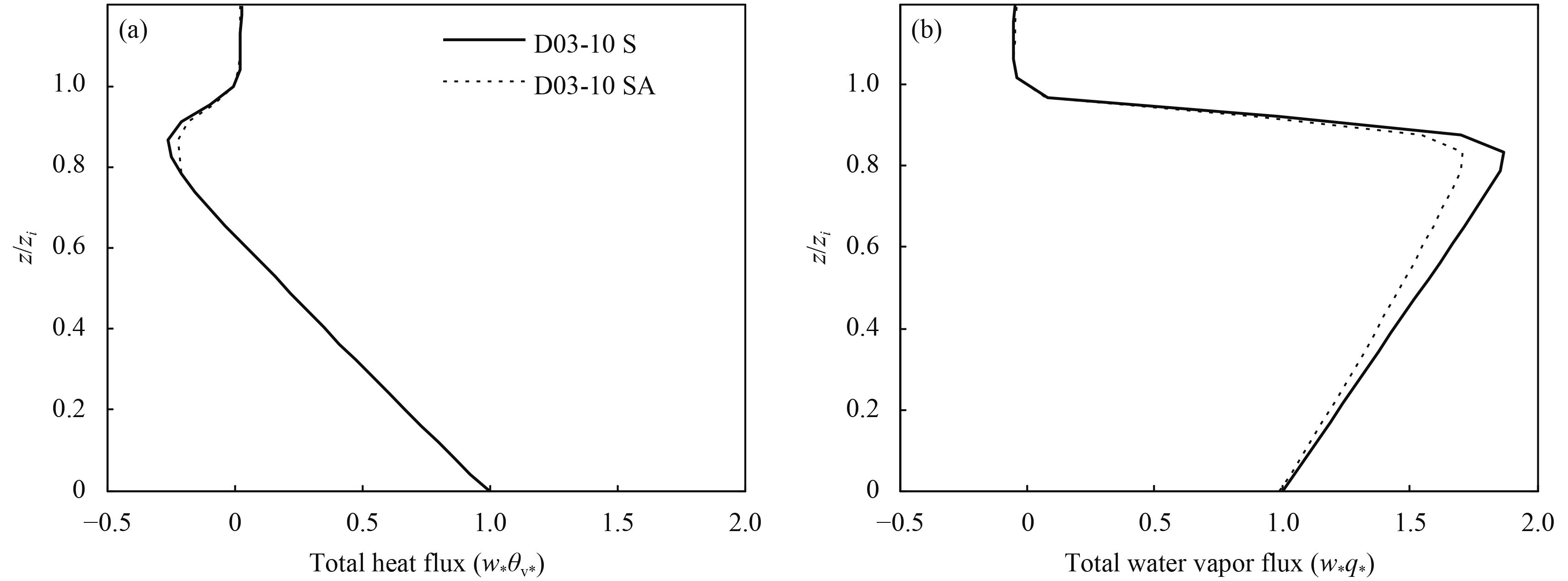Figure 12 Normalized vertical profiles of (a) total heat flux and (b) total latent heat flux from D03-10S and D03-10SA.

Moreover, the soil layer configuration has a negligible impact on total normalized sensible heat flux profile (Fig. 12a) and a small impact on total normalized latent heat flux profiles with the maximum of 1.92 in D03-10S reduced to 1.71 in D03-10SA at z/zi = 0.85 (Fig. 12b). In addition, the impact of soil layer configuration on EC, SC, and TOS sensible heat flux and SC latent heat flux is almost negligible and that on EC and TOS latent heat flux is not large (figure omitted).

5 Summary and discussion

To study the influence of strip-like patches of deserts and oases on CBL flow structures such as SC and TOS, four experiments with different soil water contents in oases using observed data were carried out, representing real-time monthly timescale variation of soil water content in oases due to irrigation in a semi-idealized mode. Phase averaging and temporal averaging were used to decompose the total vertical flux into EC turbulent flux, SC flux, and TOS flux.

SC flux is larger than TOS flux in the middle and lower CBL for both sensible and latent heat fluxes; SC latent heat flux moves downward while the other fluxes are upward; SC is the strongest and TOS is the weakest when soil water content in oases is close to saturation.

With the decrease of soil water content in oases, SC becomes weaker, TOS becomes stronger, and the atmospheric stratification over oases gradually becomes unstable. Moreover, normalized total vertical advection heat flux remains almost unchanged with the SC fraction decreasing and TOS fraction increasing, but normalized total vertical advection flux for water vapor increases significantly in the upper CBL with the SC fraction decreasing in magnitude and TOS fraction increasing.

Due to SC and TOS, horizontal domain averages of the imbalance ratios for both sensible and latent heat fluxes are large at high levels, and decreases with the decreased height. Furthermore, with soil water content in oases increasing (decreasing), the imbalance ratio becomes larger (smaller) at high levels. Because of co-existence of SC and TOS, the EC flux measured at one point may not represent the total flux, especially just after irrigation in oases.

The sensitivity experiments demonstrate that soil temperature becomes slightly higher and soil water content becomes smaller for thin soil layer, and thus, surface sensible heat flux increases while latent heat flux decreases. The soil layer configuration has only a small impact on total, EC, and TOS latent heat fluxes, but almost no impact on the rest fluxes.

Finally, different horizontal grid resolutions, horizontal winds, and averaging periods used might have influenced SC and TOS, so further investigation is needed by using different horizontal wind speeds, domain sizes, and the degree of heterogeneity. In addition, although SC and TOS have been estimated by LES over the semi-idealized strip-like patches of desert and oasis in this paper, further study is also needed over real heterogeneous land surfaces.

Appendix

The variable $[\varphi ]_{\rm p}$ is phase averaged as follows (Hadfield et al., 1991; Inagaki et al., 2006):

 ${[\varphi ]_{\rm p}}(\overline x) = \frac{1}{{{N_{\rm p}}{N_y}}}\sum\limits_{y = 1}^{N_y} {\sum\limits_{n = 1}^{N_{\rm p}} {\varphi (\overline x + n\lambda, y)} } ,$ (A1)

where $\overline x$ is the new coordinate aligned with x, which represents the position in relation to the wave cycle, $\overline x$ = x mod λ. Nx and Ny are the total number of x- and y-grids, respectively, Np is the total number of the waves in the x-direction, Np = NxΔx/λ, Δx is the grid spacing in the x-direction. The horizontal heterogeneity in the x-direction as a step function makes it possible to use phase averaging and temporal averaging to describe the vertical heat transport process of the SC. Thus, $\varphi _{\rm p}'$ denotes the phase fluctuation and represents the deviation from the phase mean at a particular model grid.

The temporal and phase average of vertical velocity (w) (positive upward) and scalar (φ) can be expressed by (Kanda et al., 2004; Steinfeld et al., 2007; Huang et al., 2008)

 $\varphi = \varphi _{\rm t}' + \overline \varphi , \quad\quad w = w_{\rm t}' + \overline w,$ (A2)
 $\varphi = \varphi _{\rm p}' + {[\overline \varphi ]_{\rm p}} , \quad w = w_{\rm p}' + {[\overline w ]_{\rm p}}.$ (A3)

Accordingly, the temporal and phase average results are written as

 $\overline {w\varphi } = \overline w \,\, \overline \varphi + \overline {w_{\rm t}'\varphi _{\rm t}'} ,$ (A4)
 ${[\overline {w\varphi } ]_{\rm p}} = {[\overline w ]_{\rm p}}{[\overline \varphi ]_{\rm p}} + {[\overline {w_{\rm p}'\varphi _{\rm p}'} ]_{\rm p}},$ (A5)

where a relationship of $\overline {w_{\rm t}'} = \overline {\varphi _{\rm t}'} = {[\overline w ]_{\rm p}} = {[\overline {\varphi _{\rm p}'} ]_{\rm p}} = 0$ is assumed. Here, $\overline {w_{\rm t}'\varphi _{\rm t}'}$ is called EC turbulent flux, and ${[\overline w ]_{\rm p}}{[\overline \varphi ]_{\rm p}}$ represents the flux induced by the SC.

Thus, to obtain the horizontal domain average of imbalance ratio [I], ([Ih] for sensible heat flux, and [Iq] for latent heat flux), Eq. (A3) is substituted into the advection term $[\overline w \overline \varphi ]$ ,

 $\begin{split}\quad[I] &= [\overline w \,\, \overline \varphi ]/[\overline {w\varphi } ]\\ &= [({\overline {w_{\rm p}' + [w]} _{\rm p}})(\overline {\varphi _{\rm p}' + {{[\varphi ]}_{\rm p}}} )]/[\overline {w\varphi } ]\\ &= ([{[\overline w ]_{\rm p}}{[\overline \varphi ]_{\rm p}}] + [{[\overline w ]_{\rm p}}\overline \varphi _{\rm p}'] + [\overline w _{\rm p}'{[\overline \varphi ]_{\rm p}}] + [\overline w _{\rm p}'\overline \varphi _{\rm p}'])/[\overline {w\varphi } ]\!\!\!\!\!\!\! \\& = ([{[\overline w ]_{\rm p}}{[\overline \varphi ]_{\rm p}}] + [\overline w _{\rm p}'\overline \varphi _{\rm p}'])/[\overline {w\varphi } ].\end{split}$ (A6)

Acknowledgments. The observation data used in this paper were kindly provided by the MUlti-Scale Observation EXpEriment on Evapotranspiration over heterogeneous land surfaces 2012 (MUSOEXE-12) under the Heihe Watershed Allied Telemetry Experimental Research (HiWATER). See their website http://westdc. westgis.ac.cn/archives/news/sciencenews/archive-144.html for details.

References
 Avissar, R., and T. Schmidt, 1998: An evaluation of the scale at which ground-surface heat flux patchiness affects the convective boundary layer using large-eddy simulations. J. Atmos. Sci., 55, 2666–2689. DOI:10.1175/1520-0469(1998)055<2666:AEOTSA>2.0.CO;2 Chu, P. C., S. H. Lu, and Y. C. Chen, 2005: A numerical modeling study on desert oasis self-supporting mechanisms. J. Hydrol., 312, 256–276. DOI:10.1016/j.jhydrol.2005.02.043 Courault, D., P. Drobinski, Y. Brunet, et al., 2007: Impact of surface heterogeneity on a buoyancy-driven convective boundary layer in light winds. Bound.-Layer Meteor., 124, 383–403. DOI:10.1007/s10546-007-9172-y Deardorff, J. W., 1972: Numerical investigation of neutral and unstable planetary boundary layers. J. Atmos. Sci., 29, 91–115. DOI:10.1175/1520-0469(1972)029<0091:NIONAU>2.0.CO;2 Deardorff, J. W., 1980: Stratocumulus-capped mixed layers derived from a three-dimensional model. Bound.-Layer Meteor., 18, 495–527. DOI:10.1007/BF00119502 Foken, T., 2008: The energy balance closure problem: An overview. Ecol. Appl., 18, 1351–1367. DOI:10.1890/06-0922.1 Foken, T., M. Mauder, C. Liebethal, et al., 2010: Energy balance closure for the LITFASS-2003 experiment. Theor. Appl. Climatol., 101, 149–160. DOI:10.1007/s00704-009-0216-8 Hadfield, M. G., W. R. Cotton, and R. A. Pielke, 1991: Large-eddy simulations of thermally forced circulations in the convective boundary layer. Part I: A small-scale circulation with zero wind. Bound.-Layer Meteor., 57, 79–114. DOI:10.1007/BF00119714 Heinemann, G., and M. Kerschgens, 2005: Comparison of methods for area-averaging surface energy fluxes over heterogeneous land surfaces using high-resolution non-hydrostatic simulations. Int. J. Climatol., 25, 379–403. DOI:10.1002/joc.1123 Huang, H. Y., and S. A. Margulis, 2010: Evaluation of a fully coupled large-eddy simulation-land surface model and its diagnosis of land–atmosphere feedbacks. Water Resour. Res., 46, W06512. DOI:10.1029/2009WR008232 Huang, J. P., X. H. Lee, and E. G. Patton, 2008: A modelling study of flux imbalance and the influence of entrainment in the convective boundary layer. Bound.-Layer Meteor., 127, 273–292. DOI:10.1007/s10546-007-9254-x Inagaki, A., M. O. Letzel, S. Raasch, et al., 2006: Impact of surface heterogeneity on energy imbalance: A study using LES. J. Meteor. Soc. Japan, 84, 187–198. DOI:10.2151/jmsj.84.187 Kanda, M., A. Inagaki, M. O. Letzel, et al., 2004: LES study of the energy imbalance problem with eddy covariance fluxes. Bound.-Layer Meteor., 110, 381–401. DOI:10.1023/B:BOUN.0000007225.45548.7a Klemp, J. B., and R. B. Wilhelmson, 1978: The simulation of three-dimensional convective storm dynamics. J. Atmos. Sci., 35, 1070–1096. DOI:10.1175/1520-0469(1978)035<1070:TSOTDC>2.0.CO;2 Klemp, J. B., J. Dudhia, and A. D. Hassiotis, 2008: An upper gravity-wave absorbing layer for NWP applications. Mon. Wea. Rev., 136, 3987–4004. DOI:10.1175/2008MWR2596.1 Li, X., G. D. Cheng, S. M. Liu, et al., 2013: Heihe watershed allied telemetry experimental research (HiWATER): Scientific objectives and experimental design. Bull. Amer. Meteor. Soc., 94, 1145–1160. DOI:10.1175/BAMS-D-12-00154.1 Liu, S. F., and Y. P. Shao, 2013: Soil-layer configuration requirement for large-eddy atmosphere and land surface coupled modeling. Atmos. Sci. Lett., 14, 112–117. DOI:10.1002/asl2.426 Liu, S. M., Z. W. Xu, W. Z. Wang, et al., 2011: A comparison of eddy-covariance and large aperture scintillometer measurements with respect to the energy balance closure problem. Hydrol. Earth Syst. Sci., 15, 1291–1306. DOI:10.5194/hess-15-1291-2011 Maronga, B., and S. Raasch, 2013: Large-eddy simulations of surface heterogeneity effects on the convective boundary layer during the LITFASS-2003 experiment. Bound.-Layer Meteor., 146, 17–44. DOI:10.1007/s10546-012-9748-z Meng, X., S. Lu, T. Zhang, et al., 2012: Impacts of inhomogeneous landscapes in oasis interior on the oasis self-maintenance mechanism by integrating numerical model with satellite data. Hydrol. Earth Syst. Sci., 16, 3729–3738. DOI:10.5194/hess-16-3729-2012 Meng, X., S. Lu, Y. Gao, et al., 2015: Simulated effects of soil moisture on oasis self-maintenance in a surrounding desert environment in Northwest China. Int. J. Climatol., 35, 4116–4125. DOI:10.1002/joc.4271 Moeng, C.-H., J. Dudhia, J. Klemp, et al., 2007: Examining two-way grid nesting for large eddy simulation of the PBL using the WRF model. Mon. Wea. Rev., 135, 2295–2311. DOI:10.1175/MWR3406.1 Niu, G. Y., Z. L. Yang, K. E. Mitchell, et al., 2011: The community Noah land surface model with multiparameterization options (Noah-MP): 1. Model description and evaluation with local-scale measurements. J. Geophys. Res., 116, D12109. DOI:10.1029/2010JD015139 Patton, E. G., P. P. Sullivan, and C.-H. Moeng, 2005: The influence of idealized heterogeneity on wet and dry planetary boundary layers coupled to the land surface. J. Atmos. Sci., 62, 2078–2097. DOI:10.1175/JAS3465.1 Raasch, S., and G. Harbusch, 2001: An analysis of secondary circulations and their effects caused by small-scale surface inhomogeneities using large-eddy simulation. Bound.-Layer Meteor., 101, 31–59. DOI:10.1023/A:1019297504109 Shao, Y. P., S. F. Liu, J. H. Schween, et al., 2013: Large-eddy atmosphere–land-surface modelling over heterogeneous surfaces: Model development and comparison with measurements. Bound.-Layer Meteor., 148, 333–356. DOI:10.1007/s10546-013-9823-0 Shen, L. D., J. N. Sun, R. M. Yuan, et al., 2016: Characteristics of secondary circulations in the convective boundary layer over two-dimensional heterogeneous surfaces. J. Meteor. Res., 30, 944–960. DOI:10.1007/s13351-016-6016-z Shen, S. H., and M. Y. Leclerc, 1995: How large must surface inhomogeneities be before they influence the convective boundary layer structure? A case study.. Quart. J. Roy. Meteor. Soc., 121, 1209–1228. DOI:10.1002/qj.49712152603 Solomon, A., M. D. Shupe, P. O. G. Persson, et al., 2011: Moisture and dynamical interactions maintaining decoupled Arctic mixed-phase stratocumulus in the presence of a humidity inversion. Atmos. Chem. Phys., 11, 10127–10148. DOI:10.5194/acp-11-10127-2011 Steinfeld, G., M. O. Letzel, S. Raasch, et al., 2007: Spatial representativeness of single tower measurements and the imbalance problem with eddy-covariance fluxes: Results of a large-eddy simulation study. Bound.-Layer Meteor., 123, 77–98. DOI:10.1007/s10546-006-9133-x Talbot, C., E. Bou-Zeid, and J. Smith, 2012: Nested mesoscale large-eddy simulations with WRF: Performance in real test cases. J. Hydrometeorol., 13, 1421–1441. DOI:10.1175/JHM-D-11-048.1 Wang, J. M., W. Z. Wang, S. M. Liu, et al., 2009: The problems of surface energy balance closure—An overview and case study. Adv. Earth Sci., 24, 705–713. DOI:10.11867/j.issn.1001-8166.2009.07.0705 Wang, R. Y., Q. Zhang, H. Zhao, et al., 2012: Analysis of the surface energy closure for a site in the Gobi Desert in Northwest China. Acta Meteor. Sinica, 26, 250–259. DOI:10.1007/s13351-012-0210-4 Yamaguchi, T., and G. Feingold, 2012: Technical note: Large-eddy simulation of cloudy boundary layer with the Advanced Research WRF model. J. Adv. Model. Earth Syst., 4, M09003. DOI:10.1029/2012MS000164 Zhang, S. W., D. Q. Li, and C. J. Qiu, 2009: A comparative study of the three land surface models in simulating the soil moisture profile. Plateau Meteor., 28, 988–996. Zhang, S. W., D. Q. Li, Y. H. Liu, et al., 2010: A comparative study of the difference algorithms for solving the soil moisture equation. J. Lanzhou Univ. Nat. Sci., 46, 46–52. DOI:10.13885/j.issn.0455-2059.2010.04.012